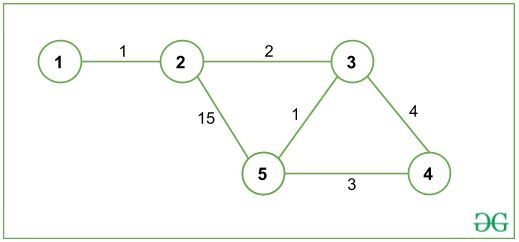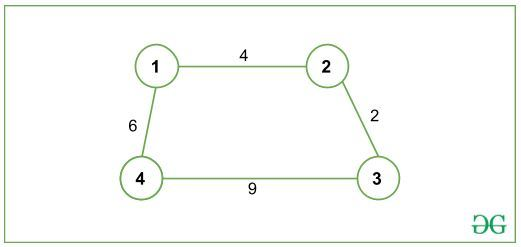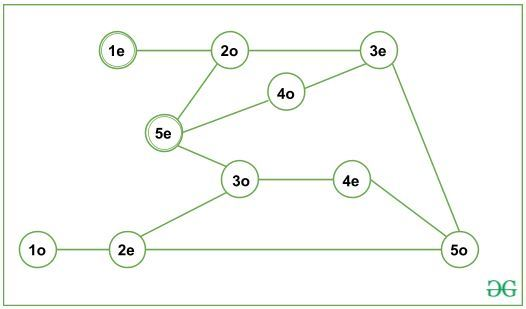# Shortest Path with even number of Edges from Source to Destination

Given an undirected graph G, the task is to find the shortest path of even-length, given 1 as Source Node and N as Destination Node. Path length refers to the number of edges present in a path (not the cost of the path).

Examples:

Input: N = 5, G is given below:Output: 10
Explanation:
All paths from 1(source node) to 5 (destination node) are:
1->2->5
Cost: 16 Length: 2(even)
1->2->3->5
Cost: 4 Length: 3(odd)
1->2->3->4->5
Cost: 10 Length: 4(even)
The shortest path is 1->2->3->5 with total cost 4, but it has an odd-length path and since we are interested in even-length paths only, the shortest path with even-length is 1->2->3->4->5, with total cost 10.

Input 2: N = 4, G is given below:Output: -1

Explanation:
There is no path of even-length from 1(source node) to 4(destination node).

## Recommended: Please try your approach on {IDE} first, before moving on to the solution.

Approach:
Create a new Graph (G’). For each node V in initial graph G, create two new nodes V_even and V_odd.

Here, V_odd will be represented as ((V * 10) + 1) and V_even as ((V * 10) + 2).
For example, if node V = 4 then V_odd = 41 and V_even = 42.

Now, for each edge (U, V) in G, add two new edges in G’, (U_even, V_odd) and (U_odd, V_even). Finally, find the Shortest path from (source_even) node to (destination_even) node using Dijkstra Shortest Path Algorithm.

For Graph given in Input 1(above), G’ can be represented as:It can observed from the graph G’ that there are only even length paths from (1_even) to (5_even). Thus, the odd-length paths get separated in G’ and the required shortest path can be obtained.

Below is the implementation of the above approach:

 `// C++ program for the above approach ` `#include ` `using` `namespace` `std; ` ` `  `const` `int` `MAXX = 10000, INF = 1e9; ` ` `  `// Adjacency List: to represent graph ` `vector > > ` `    ``adj(MAXX * 10 + 3); ` ` `  `// Distance Array: to store shortest ` `// distance to every node ` `vector<``int``> dist(MAXX * 10 + 3, INF); ` ` `  `// returns value which will ` `// represent even_x ` `int` `even(``int` `x) ` `{ ` `    ``return` `x * 10 + 2; ` `} ` `// returns value which will ` `// represent odd_x ` `int` `odd(``int` `x) ` `{ ` `    ``return` `x * 10 + 1; ` `} ` ` `  `// converting edge (a->b) to 2 ` `// different edges i.e. (a->b) ` `// converts to (1). even_a -> odd_b ` `// (2). odd_a -> even_b ` `// since, graph is undirected, so we ` `// push them in reverse order too ` `// hence, 4 push_back operations are ` `// there. ` `void` `addEdge(``int` `a, ``int` `b, ``int` `cost) ` `{ ` `    ``adj[even(a)].push_back( ` `        ``{ odd(b), cost }); ` `    ``adj[odd(a)].push_back( ` `        ``{ even(b), cost }); ` `    ``adj[odd(b)].push_back( ` `        ``{ even(a), cost }); ` `    ``adj[even(b)].push_back( ` `        ``{ odd(a), cost }); ` `} ` ` `  `// Function calculates shortest ` `// distance to all nodes from ` `// "source" using Dijkstra ` `// Shortest Path Algorithm ` `// and returns shortest distance ` `// to "destination" ` `int` `dijkstra(``int` `source, ` `             ``int` `destination) ` `{ ` ` `  `    ``/* Priority Queue/min-heap  ` `    ``to store and process ` `    ``(distance, node) */` `    ``priority_queue, ` `                   ``vector >, ` `                   ``greater > > ` `        ``pq; ` ` `  `    ``// pushing source node to ` `    ``// priority queue and dist from ` `    ``// source to source is set to 0 ` `    ``pq.push({ 0, even(source) }); ` `    ``dist[even(source)] = 0; ` ` `  `    ``while` `(!pq.empty()) { ` ` `  `        ``// U is the node at top ` `        ``// of the priority queue ` `        ``// note that pq.top().first ` `        ``// refers to the Distance ` `        ``// and pq.top().second ` `        ``// will refer to the Node ` `        ``int` `u = pq.top().second; ` `        ``pq.pop(); ` ` `  `        ``// exploring all neighbours ` `        ``// of node u ` `        ``for` `(pair<``int``, ``int``> p : ` `             ``adj[u]) { ` ` `  `            ``/* v is neighbour node of u  ` `          ``and c is the cost/weight ` `          ``of edge (u, v) */` `            ``int` `v = p.first; ` `            ``int` `c = p.second; ` ` `  `            ``// relaxation: checking if there ` `            ``// is a shorter path to v via u ` `            ``if` `(dist[u] + c ` `                ``< dist[v]) { ` ` `  `                ``// updating distance of v ` `                ``dist[v] = dist[u] + c; ` `                ``pq.push({ dist[v], v }); ` `            ``} ` `        ``} ` `    ``} ` ` `  `    ``// returning shortest ` `    ``// distance to "destination" ` `    ``return` `dist[even(destination)]; ` `} ` ` `  `// Driver function ` `int` `main() ` `{ ` `    ``// n = number of Nodes, ` `    ``// m = number of Edges ` `    ``int` `n = 5, m = 6; ` `    ``addEdge(1, 2, 1); ` `    ``addEdge(2, 3, 2); ` `    ``addEdge(2, 5, 15); ` `    ``addEdge(3, 5, 1); ` `    ``addEdge(3, 4, 4); ` `    ``addEdge(5, 4, 3); ` ` `  `    ``int` `source = 1; ` `    ``int` `destination = n; ` `    ``int` `ans = dijkstra(source, destination); ` ` `  `    ``// if ans is INF: There is no ` `    ``// even length path from source ` `    ``// to destination else path ` `    ``// exists and we print the ` `    ``// shortest distance ` `    ``if` `(ans == INF) ` `        ``cout << ``"-1"` `             ``<< ``"\n"``; ` `    ``else` `        ``cout << ans << ``"\n"``; ` ` `  `    ``return` `0; ` `} `

Output:

```10
```

Time Complexity: (E * log(V))My Personal Notes arrow_drop_upCheck out this Author's contributed articles.

If you like GeeksforGeeks and would like to contribute, you can also write an article using contribute.geeksforgeeks.org or mail your article to contribute@geeksforgeeks.org. See your article appearing on the GeeksforGeeks main page and help other Geeks.

Please Improve this article if you find anything incorrect by clicking on the "Improve Article" button below.